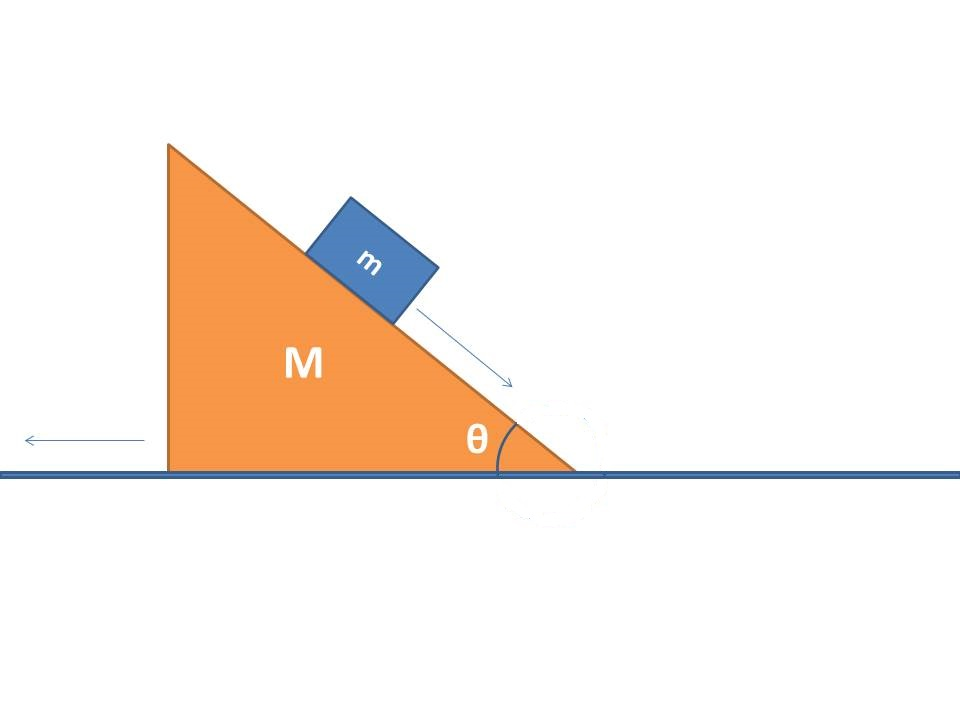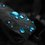# Sliding down the sliding plane.Please can anyone describe the motion of a block of mass $m$ sliding on smooth inclined plane of mass $M$ which can slide on the smooth ground. Here, $g$ is the acceleration due to gravity.Note by Shreyas S K
7 years, 1 month ago

This discussion board is a place to discuss our Daily Challenges and the math and science related to those challenges. Explanations are more than just a solution — they should explain the steps and thinking strategies that you used to obtain the solution. Comments should further the discussion of math and science.

When posting on Brilliant:

• Use the emojis to react to an explanation, whether you're congratulating a job well done , or just really confused .
• Ask specific questions about the challenge or the steps in somebody's explanation. Well-posed questions can add a lot to the discussion, but posting "I don't understand!" doesn't help anyone.
• Try to contribute something new to the discussion, whether it is an extension, generalization or other idea related to the challenge.

MarkdownAppears as
*italics* or _italics_ italics
**bold** or __bold__ bold
- bulleted- list
• bulleted
• list
1. numbered2. list
1. numbered
2. list
Note: you must add a full line of space before and after lists for them to show up correctly
paragraph 1paragraph 2

paragraph 1

paragraph 2

[example link](https://brilliant.org)example link
> This is a quote
This is a quote
    # I indented these lines
# 4 spaces, and now they show
# up as a code block.

print "hello world"
# I indented these lines
# 4 spaces, and now they show
# up as a code block.

print "hello world"
MathAppears as
Remember to wrap math in $$ ... $$ or $ ... $ to ensure proper formatting.
2 \times 3 $2 \times 3$
2^{34} $2^{34}$
a_{i-1} $a_{i-1}$
\frac{2}{3} $\frac{2}{3}$
\sqrt{2} $\sqrt{2}$
\sum_{i=1}^3 $\sum_{i=1}^3$
\sin \theta $\sin \theta$
\boxed{123} $\boxed{123}$

Sort by:

Its depand upon inclination angle

- 6 years, 10 months ago

Transitional

- 6 years, 10 months ago

the best method to solve or to understand this type of motion is psuedo accerlation

- 6 years, 11 months ago

second equation will be : mg = ma sin (theta) + N cos (theta)

- 6 years, 11 months ago

it undergoes a translational and linear motion

- 6 years, 11 months ago

Displacement of centre of mass will be vertically downward.

- 6 years, 11 months ago

Nsin[theta]=MA mgsin[theta]=MA A=mgsin[theta]/M THEN ACCORDING TO NON INERTIAL FRAME NET ACCELERATION OF m BLOCK IS A+a where a=gsin[theta] a[net]=mgsin[theta]/M+gsin[theta] a[net]=gsin[theta][m+M/M] i think this would be the correct ans for the question

- 6 years, 11 months ago

Well, I wish I could help, and also couldn't give you a diagram. First, we must make an agreement to the symbol and the sign, the positive sign and negative sign. Let the block mass m, an inclined plane M, an acceleration for block a, and an acceleration for M is A. We could look the entire objects or it's called a system. The equation will be Sigma Fx=ma, since it's m and a are system we could write as sigma Fx=(m+M)(a cos theta-A). why it should a cos theta? look at the picture, there's an arrow when the small mass are sliding, you could divide it into a cos theta and a sin theta (into a resultant) then you choose the angle is which have a relation to the plane. look again at the equations, it's said Fx, so we should look the acceleration which related to x-axis. then continue to the force, the only force which works on the plane is w sin theta, because there's no other force like friction (because the plane is smooth. so, comes the equation, mg sin theta=(m+M)(a cos theta-A). because you ask the motion of the block, so, a=[mg sin theta/m+M +A]1/cos theta.

- 6 years, 11 months ago

If we take wedge frame of reference, then there is a pseudo acceleration on the block of mass m to the right. Then use Normal and 'mg' downwards to find the acceleration.

- 6 years, 11 months ago

equations as Aveneil mentioned

- 6 years, 11 months ago

gsino. Other wise free fall

- 6 years, 11 months ago

I guess translatory motion

- 6 years, 11 months ago

dude if plane is friction less then its acceleration will a=gsin(thita) so simple

- 6 years, 12 months ago

f=ma+mg

- 6 years, 12 months ago

because of the gravity why its sliding and the shape of the triangle to

- 6 years, 12 months ago

Khan Academy can very well explain you this..

- 7 years ago

I cannot paste a diagram here but i hope you understand. Take a normal force $N$ perpendicular to $m$. I will be solving the sum in the frame of reference of ground, so no pseudo force is required.

Let accelerations of $m$ be $a( wrt. M)$ and that of $M$ be $A$.

Note that the acceleration of $m$ wrt. ground in horizontal direction is $(a cosθ - A)$. In vertical direction, it is $a sinθ.$

Writing equations,

$Nsinθ = MA$

$Mg - N cos θ = ma~sinθ$

$Nsinθ = m ( acosθ - A)$

Solving,

$a = \dfrac{(M+m)gsinθ}{M + msin^{2}θ}$

$A = \dfrac{mgsinθcosθ}{M + msin^{2}θ}$

- 7 years ago

i dnt thnk so the horizontal acceleration is acos[tita]-A instead it is acos[tita]+A .as its a non inertial frame so pseudo force acts in opposite direction to the motion of the bigger block

- 6 years, 11 months ago

Thank you very much

- 6 years, 11 months ago

if we consider this system wrt wedge then we should take the pseudo force as it is in non inertial frame but you didnt considered it.....why????

- 6 years, 12 months ago

See my solution again, I have mentioned that i am solving it wrt ground frame!

- 6 years, 12 months ago

got it thanks .........

- 6 years, 12 months ago